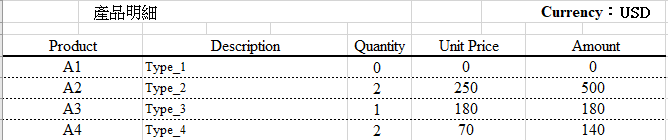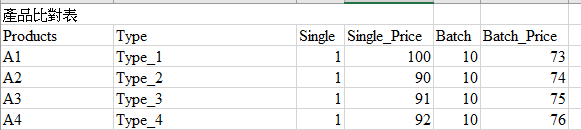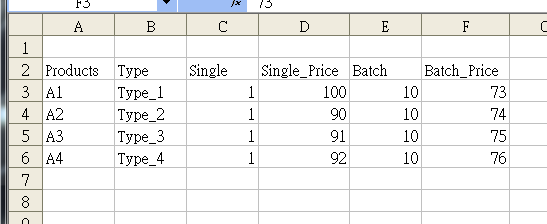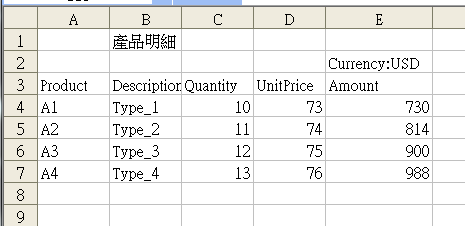#0

## 求解:excel 查表，比對，判斷與傳回對應的值ekkusu iT邦新手 5 級 ‧ 2019-12-10 17:10:11 檢舉
=if(Quantity<Batch,Single_Price,Batch_Price)

new_p iT邦新手 5 級 ‧ 2019-12-11 01:55:12 檢舉
to 海綿寶寶，是我之前的表拿來表示，我沒更改，抱歉

### 2 個回答

1new_p iT邦新手 5 級 ‧ 2019-12-11 23:14:19 檢舉

0Sheet1中部份公式如下

``````D4=IF(C4<VLOOKUP(A4,Sheet2!\$A\$3:\$F\$6,5),VLOOKUP(A4,Sheet2!\$A\$3:\$F\$6,4),VLOOKUP(A4,Sheet2!\$A\$3:\$F\$6,6))
D5=IF(C5<VLOOKUP(A5,Sheet2!\$A\$3:\$F\$6,5),VLOOKUP(A5,Sheet2!\$A\$3:\$F\$6,4),VLOOKUP(A5,Sheet2!\$A\$3:\$F\$6,6))
D6=IF(C6<VLOOKUP(A6,Sheet2!\$A\$3:\$F\$6,5),VLOOKUP(A6,Sheet2!\$A\$3:\$F\$6,4),VLOOKUP(A6,Sheet2!\$A\$3:\$F\$6,6))
D7=IF(C7<VLOOKUP(A7,Sheet2!\$A\$3:\$F\$6,5),VLOOKUP(A7,Sheet2!\$A\$3:\$F\$6,4),VLOOKUP(A7,Sheet2!\$A\$3:\$F\$6,6))
``````